Absolute Value 3
Set 3 (10 Questions)
Understand the meaning of the absolute value of a number, interpret the absolute value as the distance of the number from zero on a number line; and determine the absolute value of real numbers.

From Mr. Anker Tests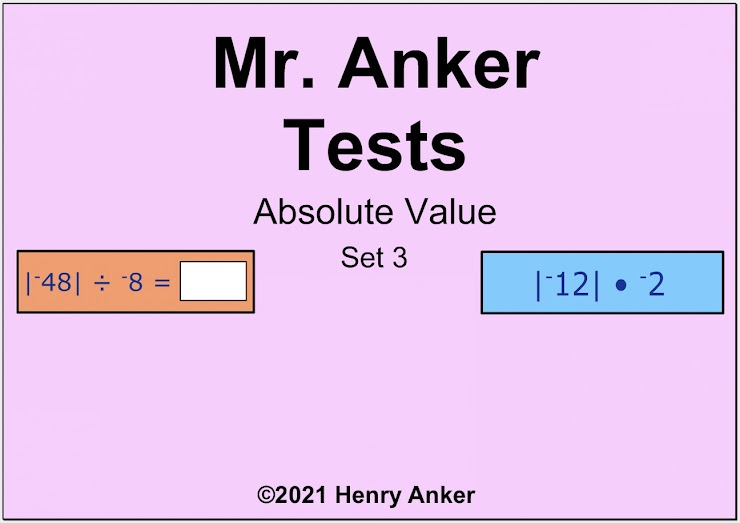Absolute Value Help Slide 1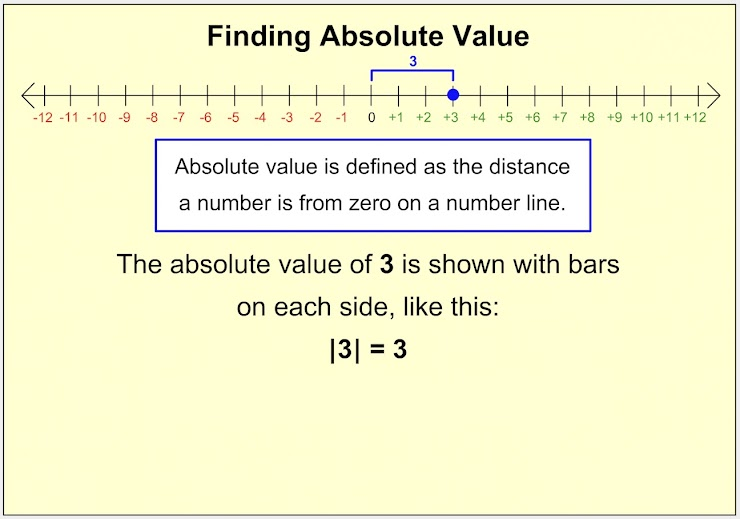Absolute Value Help Slide 2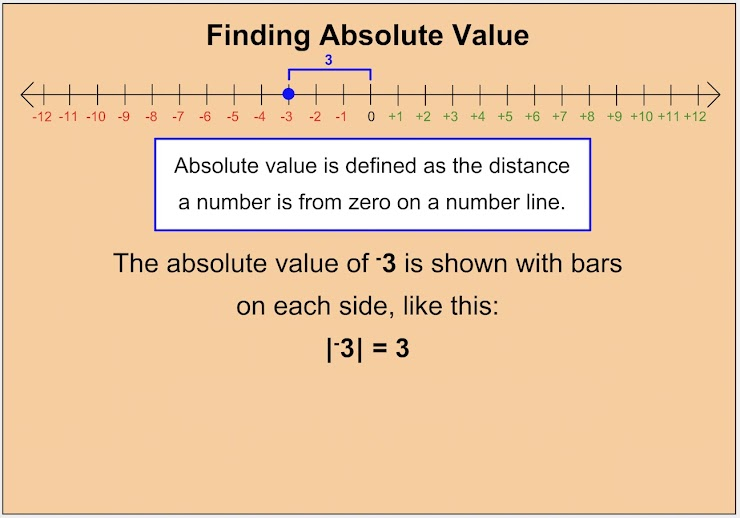Absolute Value Help Slide 3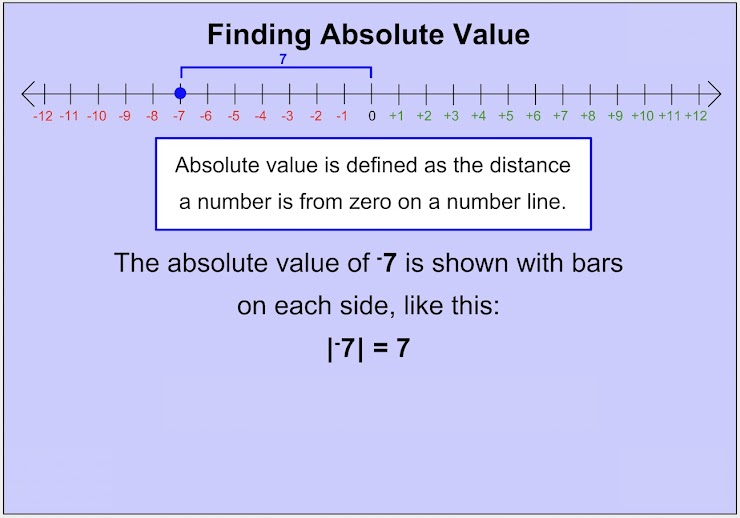Absolute Value Help Slide 4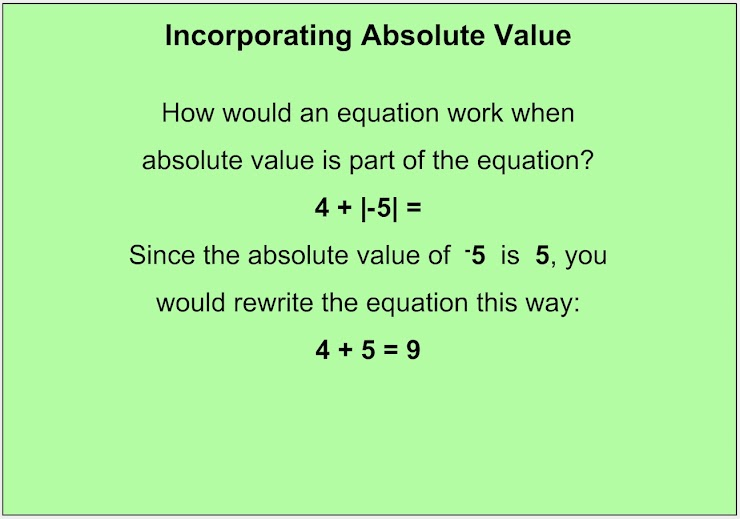Absolute Value Help Slide 5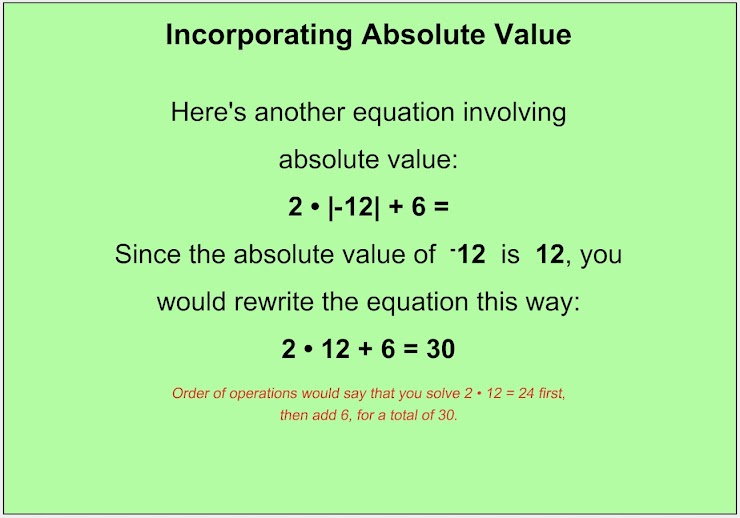Absolute Value Help Slide 6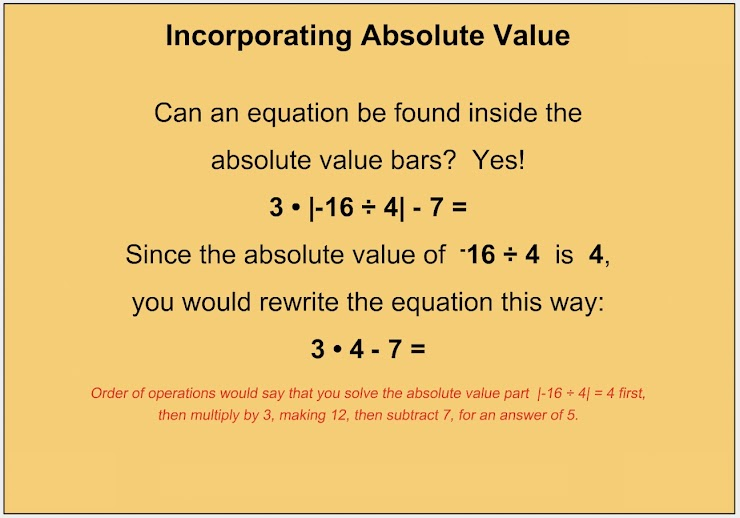Absolute Value Help Slide 7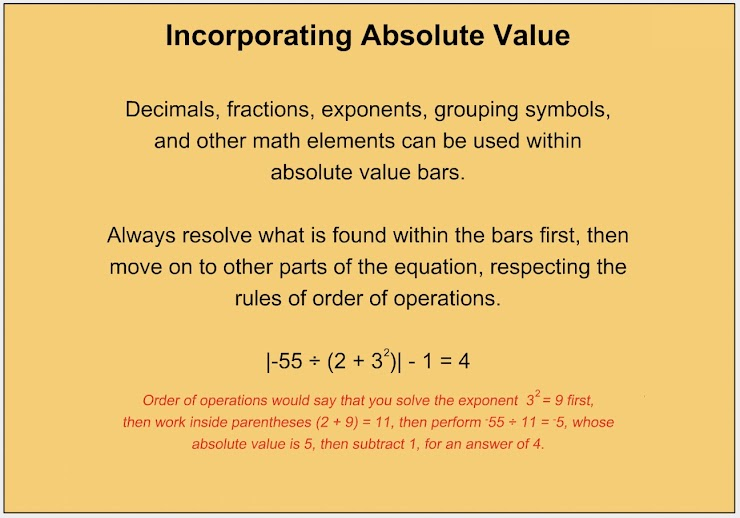Number Line (Absolute value shows the distance from zero.)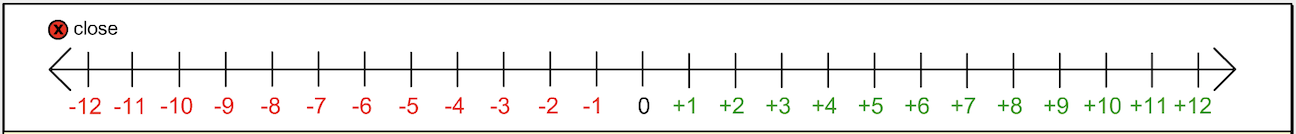1.  Which of the choices below is the correct answer to this division fact? *
1 point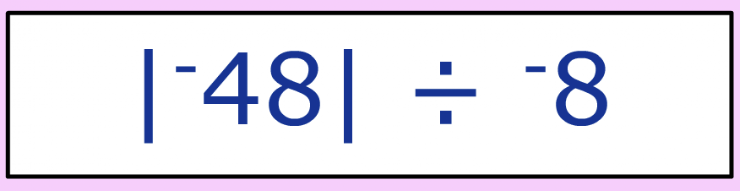2.  Type your answer to this equation on the line below. *
1 point3.  Use the dropdown menu to make this statement true:  -3 is __________ |-1 + -2|. *
1 point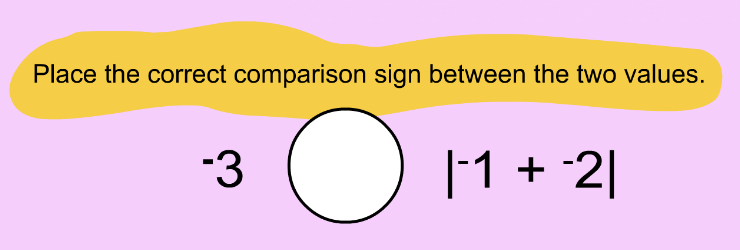4. Which of the choices below is the correct answer to this multiplication fact? *
1 point5.  Type your answer to this equation on the line below. *
1 point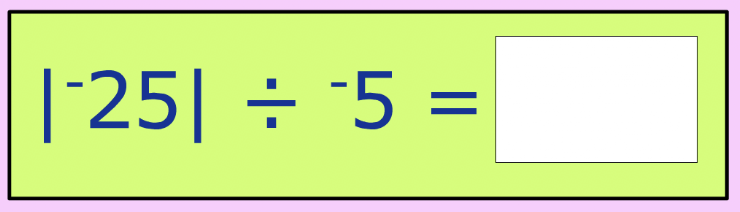6. Use the dropdown menu to make this statement true:  |-11| + -4 is __________ |-21| ÷ 3 *
1 point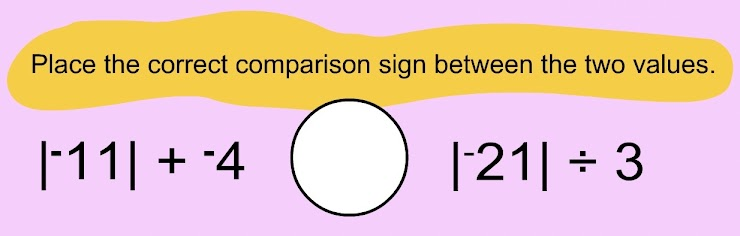7.  Which of the choices below is the correct answer to this addition fact? *
1 point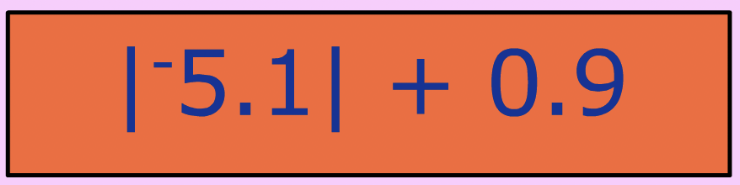8.  Type your answer to this equation on the line below. *
1 point9.  Use the dropdown menu to make this statement true:  -3 is _____ -|-3| *
1 point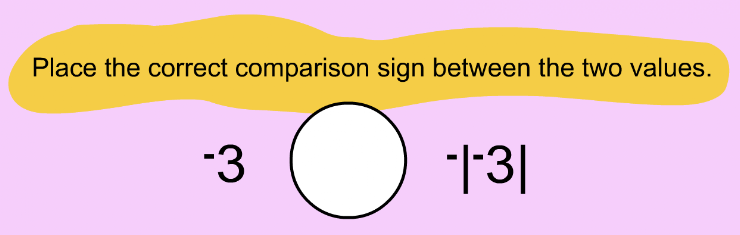10.   Which of the choices below is the correct answer to this division fact? *
1 point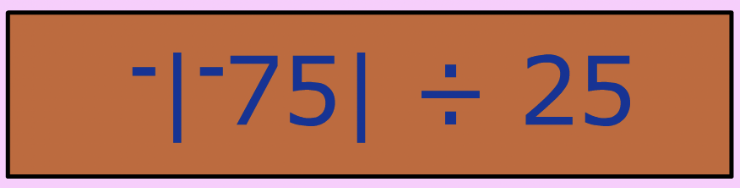Submit
Clear form